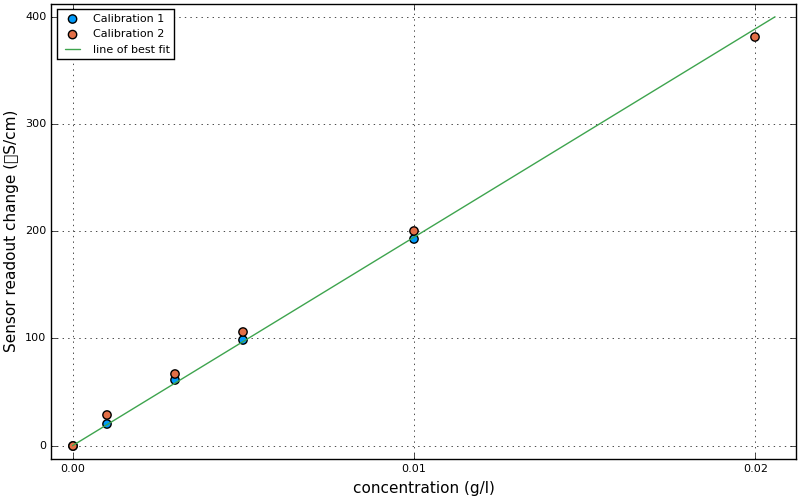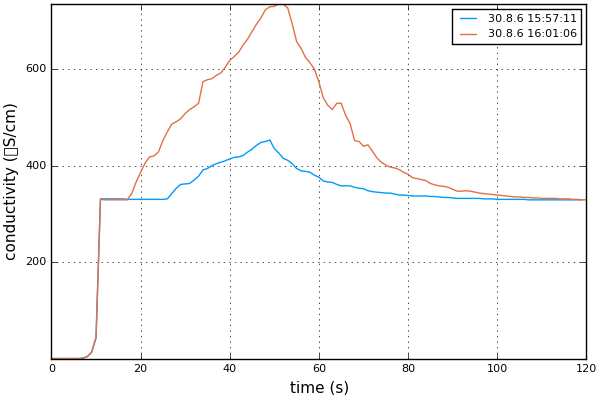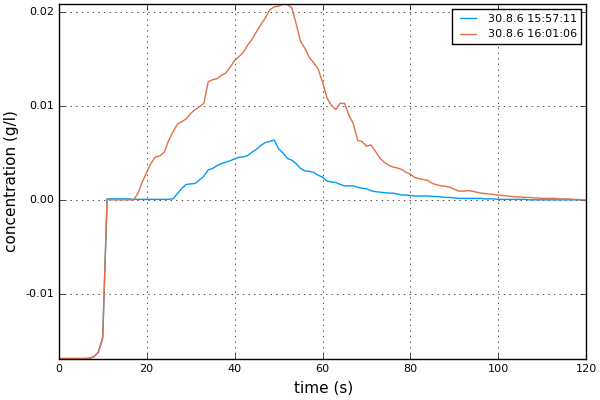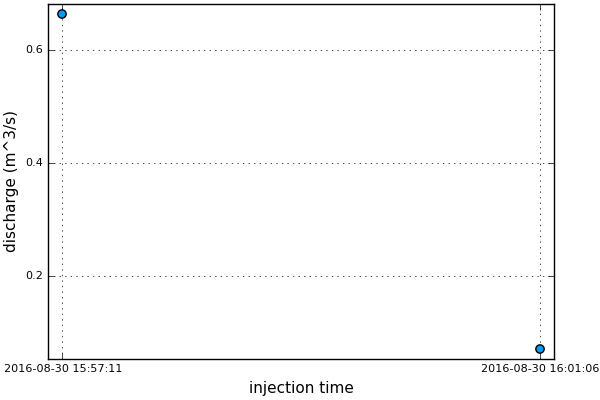# Evaluation of salt dilution stream gauging¶

This notebook guides you through the evaluation of the salt dilution stream gauging for the glaciology field course. The idea is that you modify the code in this notebook such that your experiments are evaluated.

## Technical instructions¶

The notebook is a Jupyter notebook (formerly iPython notebook) which allows combining notes (in markdown), codes and plots. For documentation see here, but you should be able to get by with the documentation I provide below.

To get the notebook running (what you're looking at right now is probably the non-inteactive html-rendered version of the notebook) do:

• open a terminal and type: cd fieldcourse/surface-hydrology/ + enter, julia + enter
• once started type using IJulia + enter and notebook() + enter (this will take a few seconds).
• this should open a file-browser dialog in your internet-browser (if it doesn't open a browser and navigate to localhost:8888, by clicking the link). Click on Salt-dilution.ipynb which should open a new tab. The tab should look like the html-version of the notebook you looked at so far but is now fully functional, i.e. you can edit it and exectue code.

If you want to run this on your own computer you'll have to install Julia 0.4.6 and install the packages IJulia, NBInclude, Plots, PyPlot, LsqFit by running at the Julia prompt Pkg.add("IJulia") etc.

The important notebook commands are:

• clicking or double-clicking into a cell allows you to edit it.
• pressing shift+enter evaluates it: for a cell like the one you are reading now it renders the markdown, for code cells it evaluates the code and prints/displays the output of the last line of code. Use a ; to supress the output.
• variables and functions defined in one cell will be available for use in cells evaluated afterwards (irrespective of whether the cell is above or below).
• if you change a code-cell and re-evaluate it, the cells depending on its variables are not evaluated again automatically.
• somtimes it is good to re-start the computation and re-evaluated all cells. Go to drop down menu Kernel -> Restart and run all
• there is no undo! E.g. if you mark the contents of a cell and press backspace, it's gone, bye-bye! Thus save often (disk-icon top left). It should also auto-save but still, save often. To revert to the last save go to menu File -> Revert to Checkpoint (this is as close to undo as it gets!).
• To insert a new, empty cell got to the menu Insert
• To change the type of the cell use the drop-down menu which displays Code by default.
• To export the notebook as a pdf, html, etc. got to drop-down menu File -> Download as

## Julia¶

This notebook uses the Julia programming language (version 0.4.6), which is a new technical programming language. Its syntax is fairly close to Matlab, at least for easy stuff, to which I'll stick to here. Notable differences to Matlab are:

• indexing is done with [], e.g. a.
• functions can be defined in-line and don't need their own m-file

Differences to python:

• indexing starts at 1
• indentation does not matter, instead blocks/functions, etc are closed with end.

Documentation for Julia can be found here. If you're interested to get started with Julia (which I can reccomend but please after this course!) have a look at this tutorial, with materials here.

## Let's get started¶

First load the plotting library Plots.jl and the helper functions in the notebook Salt-dilution-helper-functions.ipynb (If you want to look at it, it's in the same directory as this file. You can open it by clicking it in the file-browser tab. Clicking here should also open it. Once opened you can edit it too.)

In :
# Import the plotting package "Plots" and makes its functions available.
using Plots

# Include a notebook-file which contains misc. helper functions
# (in the same directory as this notebook).  You can open it by clicking on it in the file-browser.
using NBInclude
nbinclude("Salt-dilution-helper-functions.ipynb");


## Calibration data¶

You performed several calibrations. Adapt below cell to contain your calibration results:

• the bucketsize is the size of the bucket/bottle in which you preformed the calibration (in liters).
• the solution is the concentration of the calibration solution (in g/l).
• The cali1, etc., variables should contain total $ml$ calibration solution added (first column) and readout in $μS/cm$ (second column).
In :
# UPDATE these two variables!!!
bucketsize = 1.0 # calibration bucket size in liters
solution = 1.0 # calibration solution concentration (g/l)

# total calibration ml solution vs sensor readout (μS/cm)
# NOTE: this is bongus data.  Yours will look quite differenly!

# first calibration on 30.8.2016 at 15:34
cali1 = [ 0 331 # First row needs to be the background reading!
1 351 # Note, that background reading will probably be much different for you
3 392
5 430
10 524]
# second calibration 31.8.2016
cali2 = [ 0 320
1 349
3 387
5 426
10 520
20 701]
# more calibarations
# cali3 = ...
;

In :
"""
Converts ml added to bucket to a concentration (g/l == kg/m^3).

Input:

- ml -- how many mililiters were added
- solution -- the concentration of the calibration solution (kg/m^3 == g/l)
- bucketsize -- the size of the bucket/bottle to which the solution was added (l)

Output:

- concentration (kg/m^3 == g/l)
"""
function ml_to_concentration(ml, solution, bucketsize)
mass = ml/1e3 * solution # salt mass added to bucket (g)
return mass/bucketsize # concentration in g/l (== kg/m^3)
end
# For example, convert cali1[:,1] to concentration (g/l):
ml_to_concentration(cali1[:,1], solution, bucketsize)

Out:
5-element Array{Float64,1}:
0.0
0.001
0.003
0.005
0.01 

What we are really after is a function which tells us the concentration for a given sensor readout. This is done with the fit_calibration function which is contained in the extra file Salt-dilution-helper-functions.jl (You can treat it as a black-box but feel free to look at it too). Running this returns us just such a function, by fitting a straight line through the data:

$f(x) = ax$

where $x$ is difference between the readout and the readout at 0 concentration, and $a$ is the parameter to fit. It will also tell you how good the fit is by giving the error on a. The error should be "reasonably" small.

In :
# Fit a straight line through the calibrations
# (executing this the first time will take ~10s, a "In [*]" on the side of the cell indicates that julia is
#  doing calculations)
#
delta_readout2conc = fit_calibration(bucketsize, solution, cali1, cali2);

Estimated linear fit: f(delta_readout) = a*conc with
a = 5.1435756708442425e-5±1.0953578016663672e-6



With delta_readout2conc we now have a function which converts the sensor readout (above background) to a salt concentration, just what we need further down! Let's have a look at how well the line fits the data by plotting it:

In :
## Plot the calibration points:
# scatter plots (x,y) points
scatter(ml_to_concentration(cali1[:,1], solution, bucketsize), cali1[:,2]-cali1[1,2],
xlabel="concentration (g/l)", ylabel="Sensor readout change (μS/cm)",
label="Calibration 1", legend=:topleft, size=(800,500))
# scatter! plots (x,y) points into the current plot (as opposed to make a new one)
scatter!(ml_to_concentration(cali2[:,1], solution, bucketsize), cali2[:,2]-cali2[1,2],
label="Calibration 2")
# add more plots of calibrations here by copy-pasting-adapting the cali2 plot...

## Now plot the line of best fit:
# plot! plots all sorts of things, but here a line.  Again the !-variant adds it to the existing plot

# (executing this the first time will take ~20s because the plotting package needs to initialize)

[Plots.jl] Initializing backend: pyplot

Out:That's the calibrations done. If you got several quite different calibration results, say from the proglacial stream and the supraglacial stream, then use only their respecive calibrations for a set of traces.

The datafile has the format:

Device;Device serial;ID;Date/Time;Value;Unit;Mode;Value2;Unit2;Mode2;Measurement;Calibration;Additional;Sensor;Sensor serial;User
Multi 3630; 16231200;2;12.08.2016 13:36:58;0.1;µS/cm;Cond;25.6;°C;Temp;;;C = 0.475 1/cm   Tref25   nLF;TetraCon 925-P; 16210277;
...

We're interested in columns: time, conductivity (µS/cm), and temperature (potentially useful to check that the sensor was in the water). The loading is implemented in the function read_conductivity_data in the notebook Salt-dilution-helper-functions.ipynb, which was loaded above. Again you can treat that function as a black-box:

In :
ts, readouts, temps = read_conductivity_data("AD281106_example.CSV")
traces = split_conductivity_data(ts, readouts, temps, 1.0)

# if there are several files:
# traces2 = split_conductivity_data(ts, readouts, temps, 1.0)
# traces = vcat(traces, traces2)

Out:
2-element Array{Any,1}:
([0.0,1.0,2.0,3.0,4.0,5.0,6.0,7.0,8.0,9.0  …  110.0,111.0,112.0,113.0,114.0,115.0,116.0,117.0,118.0,119.0],2016-08-30T15:57:11,[0.1,0.1,0.1,0.1,0.1,0.1,0.1,1.1,3.8,12.9  …  329.0,329.0,329.0,329.0,329.0,329.0,329.0,329.0,329.0,329.0],[22.6,22.6,22.6,22.6,22.6,22.6,22.5,22.5,22.6,22.6  …  21.7,21.7,21.7,21.7,21.7,21.7,21.7,21.7,21.7,21.7])
([0.0,1.0,2.0,3.0,4.0,5.0,6.0,7.0,8.0,9.0  …  111.0,112.0,113.0,114.0,115.0,116.0,117.0,118.0,119.0,120.0],2016-08-30T16:01:06,[0.2,0.2,0.2,0.2,0.2,0.2,0.2,0.2,3.9,13.4  …  332.0,332.0,332.0,331.0,331.0,331.0,330.0,330.0,329.0,329.0],[21.7,21.7,21.7,21.7,21.7,21.7,21.7,21.7,21.7,21.7  …  21.6,21.6,21.6,21.6,21.6,21.6,21.6,21.6,21.6,21.6])

Aside: Note that for most functions, including the ones in the Salt-dilution-helper-functions.ipynb file, help can be printed by typing ?read_conductivity_data (as the only thing in a cell):

In :
?split_conductivity_data

search: split_conductivity_data


Out:

Splits up the time series returned by read_conductivity_data into individual traces. The output is a list of traces:

traces = [(t1, tstart1, readout1, temp1), (t2, tstart2, readout2, temp2), etc]

where t1 is the times in seconds after recoding started, tstart1 is the date-time of the start of the recording, readout1 is the conductivity readout and temp1 is the sensor temperature.

Thus to access the times of the second trace do traces, and to get the corresponding sensor readout traces.

In :
# running this the first time will take ~10s because it initializes the plotting facilities
p = plot()
for (t, tstart, readout, temp) in traces
xlabel="time (s)", ylabel="conductivity (μS/cm)",
label=Base.Dates.format(tstart, "d.m.y HH:MM:SS") )
end
p

Out:This is bongus data (produced by holding the conductivity sensor into my glas, then adding salt and then diluting). Your breakthrough curve should look like much smoother!

In :
# Now plot the concentrations
p = plot()
for (t, tstart, readout, temp) in traces
xlabel="time (s)", ylabel="concentration (g/l)",
label=Base.Dates.format(tstart, "d.m.y HH:MM:SS") )
end
p

Out:## Determine discharge of one dilution experiment¶

Now that we have the data loaded, we can determine the discharge. The concentration $C$ times the (unknown) stream discharge $Q$ gives the salt mass flux. Integrate this over the the whole breakthrough curve to get the injected mass $M$:

$M = Q \int C \, d t$

This assumes that $Q$ is constant during the salt passage (a good assumption). Solve for the unknow $Q$:

$Q = \frac{M}{\int C \, d t}$.

This is what we calculate here.

In :
"""
Integrates the concentration time series.

Input:

- t -- times
- t1, t2 -- integrate from t1 to t2.  Note that the concentration should be
close to zero at both t1 and t2 as we want to integrate over the whole curve.
- conc -- concentration time series (convert conductivity with f_readout2conc
to a concentration)

Output:

- integrated concentration (g s/l) == (kg s/ m^3)
"""
function integrate_concentration(t, conc, t1=0.0, t2=Inf)
inds = findfirst(t.>=t1):findlast(t.<=t2)
dt = t-t
out = sum(conc[inds]*dt) # approximate the integral by a sum
if out==0
error("Concentration integrates to zero!  Maybe to high temp_threshold?")
end
return out
end

"""

Input:
- mass -- mass of salt injected

Optional:
- t1,t2 -- integration limits (otherwise the whole series is integrated)
- delta_readout2conc -- if you got several delta_readout2conc functions, then
pass it in explicitly.

Output:
- discharge Q (m^3/s)
"""
i1, i2 = findfirst(t.>=t1), findlast(t.<=t2)
return mass/integrate_concentration(t, conc, t1, t2)
end;


Now let's use this to finally calculate the discharge:

In :
# You need to update this to reflect your salt injection masses:
masses = [0.1, 0.05]
# Integration boundaries.  If the sensor was submerged before logging started, then
# you can probably delete t1s and t2s (also in below loop).  If not, like in the fake-examples below,
# the boundaries need to be set to avoid integrating the negative concentrations from 0-15s.
t1s = [15, 15]
t2s = [Inf, Inf]
# Store the times and discharges
ts = DateTime[]
Qs = Float64[]  # this will contain the discharges
for i = 1:length(traces)
t, tstart, readout, temp = traces[i]
Q = calcQ(t, readout, masses[i], t1s[i], t2s[i]) # in m^3/s
println("Discharge for experiment $i is$(Q*1000) l/s")
push!(Qs, Q)
push!(ts, tstart)
end;

Discharge for experiment 1 is 664.6744672548527 l/s
Discharge for experiment 2 is 71.16298743486254 l/s

In :
# plot the discharge vs time:
scatter(ts, Qs, label="", xlabel="injection time", ylabel="discharge (m^3/s)")

Out:## Store output in text files¶

In :
# store discharge and time in a csv file
out = hcat(ts, Qs)
writecsv("discharge.csv", out) # this will write the date-time in ISO format, which should be easy to read-in

# if you prefer this writes it as days since some date:
out = hcat(datetime2julian(ts), Qs)
writecsv("discharge-julian.csv", out)


# More stuff to do¶

Try to find a stage-discharge relation: fit a line (probably not staight) through the (stage, Qs) points (if you want to do this in Julia, look at the fit_calibration function in the helper-file). Where stage are the stage measurements you took simultaneously with the salt dilution. Also try using the cross section instead of the stage.

Calculate discharge using the velocity x cross-sectional area method (if you managed to get those measurements). How does it compare to the discharge from salt dilution?

Calculate the melt which occured in the moulin catchment from the ablation measurement. How does it compare to the measured discharge? Is there a lag? If there is a lag, can you fit a linear storage model: https://en.wikipedia.org/wiki/Runoff_model_(reservoir)?

In [ ]: